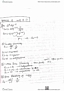ECON 212 Quiz: ECON 212quizQuiz 3 Section 002 Solution

8 views4 pages
School
Department
Course
Professortaupeweasel79 and 78 others unlocked32
Verified Note
32 documents

Document Summary

Econ 212 - winter 2017 - section 002 - quiz 3 - solution. 1. a) (3 points) peter consumes goods x and y. His utility function is u (x, y) = x y3/4. The price of good x is px, and the price of good y is py. Derive peter"s demand functions for good x and good y. The optimal consumption bundle occurs when m rsx, y = px. Px y into the budget constraint px x + py y = i to obtain: 3 py y + py y = (cid:0) 4. 3 py y = i = y = 3. Therefore, the demand functions are: x = 4. Calculate peter"s optimal demand for each good if px = 8, py = 9, and. I = 840, using the demand functions that you derive above. (ii. ) As you go through the solution below, you can refer to the graph above in order to help you understand the solution. (i. )